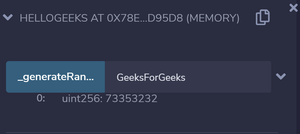# What is Hashing in Solidity?

A cryptographic hash function is an algorithm that takes an arbitrary amount of data as input and produces the enciphered text of fixed size. Even a slight change in the input gives a completely different output.

Solidity provides the following cryptographic functions:

Ethereum uses Keccak for hashing which is similar but not the same as SHA_256. For proof of work, it uses a custom scheme called ethash which is designed to be ASIC-resistant.

Example: In the below example, a smart contract is created to take a string as input and give an 8 digit hash as an output.

## Solidity

 `// pragma version ` `pragma solidity ^0.6.6;  ` ` `  `// Creating a contract ` `contract` `helloGeeks  ` `{ ` `    ``// We want hash to be of 8 digits  ` `    ``// hence we store 10^8 which is  ` `    ``// used to extract first 8 digits  ` `    ``// later by Modulus  ` `    ``uint hashDigits = 8; ` `     `  `    ``// Equivalent to 10^8 = 8 ` `    ``uint hashModulus = 10 ** hashDigits;  ` ` `  `    ``// Function to generate the hash value ` `    ``function _generateRandom(string memory _str)  ` `        ``public` `view returns (uint)  ` `    ``{ ` `        ``// "packing" the string into bytes and  ` `        ``// then applying the hash function.  ` `        ``// This is then typecasted into uint. ` `        ``uint random =  ` `             ``uint(keccak256(abi.encodePacked(_str))); ` `              `  `        ``// Returning the generated hash value  ` `        ``return` `random % hashModulus; ` `    ``} ` ` `  `}`

Input:

```GeeksForGeeks
```

Output:My Personal Notes arrow_drop_upCheck out this Author's contributed articles.

If you like GeeksforGeeks and would like to contribute, you can also write an article using contribute.geeksforgeeks.org or mail your article to contribute@geeksforgeeks.org. See your article appearing on the GeeksforGeeks main page and help other Geeks.

Please Improve this article if you find anything incorrect by clicking on the "Improve Article" button below.

Article Tags :

Be the First to upvote.

Please write to us at contribute@geeksforgeeks.org to report any issue with the above content.